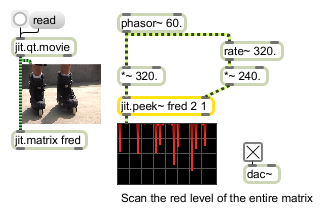# jit.peek~

Read matrix data as an audio signal

## Description

The jit.peek~ object reads the value of one plane of a matrix cell at the position specified by the signal inputs. This value is output as a signal. The object arguments are [matrix_name] [dim_inputcount] [plane] .

## Arguments

Name Type Opt Description
matrix_name The named matrix to access (default = none)
dim_inputcount The number of dimensions in the matrix (default = 2)
plane The plane in a cell whose value is output (default = 0)

## Messages

 signal All inlet: Signal values arriving in these inlets specify the dimensional location of the data to be stored for the plane specified. A signal inlet is provided for each dimension specified by the dim_inputcount argument (i.e., jit.peek~ bigcube 3 has 3 inlets -- one for each of the 3 dimensions of the matrix).

## Attributes

Name Type g/s Description
matrix_name symbol The named matrix to access (default = none)
plane int The plane in a cell whose value is output (default = 0)
interp int Interpolation flag (default = 0)
normalize int Normalizated input coordinates flag (default = 0)

## Examples